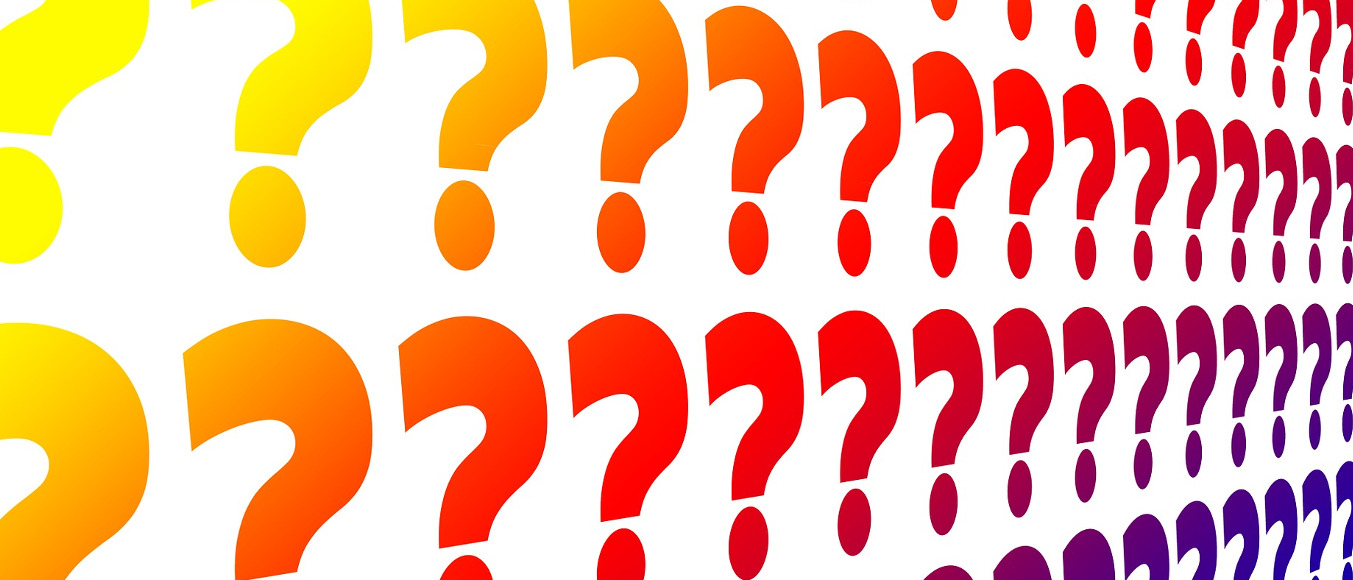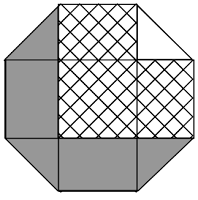# Can you solve these puzzles?

While we work on issue 2Issue 2 of Chalkdust magazine will be released on Tuesday 6th October, in just under 5 weeks’ time. While we work on writing, editing and designing the content for the magazine, here are a few puzzles to keep you entertained…

### Make the SumsSource: mscroggs.co.uk
Put the digits 1 to 9 (using each digit exactly once) in the boxes so that the sums reading across and down are correct. The sums should be read left to right and top to bottom ignoring the usual order of operations. For example, 4+3×2 is 14, not 10.

### Unit OctagonSource: UKMT Junior Mathematics Challenge 2014
The diagram shows a regular octagon with sides of length 1. The octagon is divided into regions by four diagonals. What is the difference between the area of the hatched region and the area of the region shaded grey?

### Reverse Bases

Source: mscroggs.co.uk
Find two digits $a$ and $b$ such that $ab$ in base 10 is equal to $ba$ in base 4.
Find two digits $c$ and $d$ such that $cd$ in base 10 is equal to $dc$ in base 7.
Find two digits $e$ and $f$ such that $ef$ in base 9 is equal to $fe$ in base 5.

The answers to these puzzles will appear here on Sunday at midday (GMT).Matthew Scroggs is a postdoctoral researcher in the Department of Engineering at the University of Cambridge working on finite and boundary element methods. His website, mscroggs.co.uk, is full of maths.
@mscroggs    mscroggs.co.uk    + More articles by Matthew